Computer Science

# Homework 1b — Functions

## 1. One Tree

You are given some code in `one_tree.py`. Bit starts in a 5x4 world:Bit should draw a tree: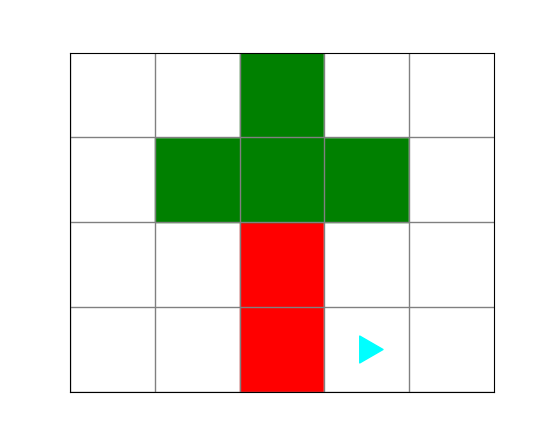In the code we have given you, we have decomposed the problem for you:

``````from byubit import Bit

def move_to_tree(bit):
""" Moves to the trunk """
pass

def draw_trunk(bit):
""" Draws the trunk (two red squares)  """
pass

def draw_branches(bit):
""" Draws the branches """
pass

def go_back_down(bit):
""" Moves back down to the ground, below the right-most branch, facing right. """
pass

@Bit.empty_world(5, 4)
def one_tree(bit):
move_to_tree(bit)
draw_trunk(bit)
draw_branches(bit)
go_back_down(bit)

if __name__ == '__main__':
one_tree(Bit.new_bit)``````

We want you to see an example of how each function does one small thing.

You should be able to draw the tree by filling in each of these four functions, without using any glue code.

## 2. Trees

You are given some code in `trees.py`. Bit starts in a 13x4 world: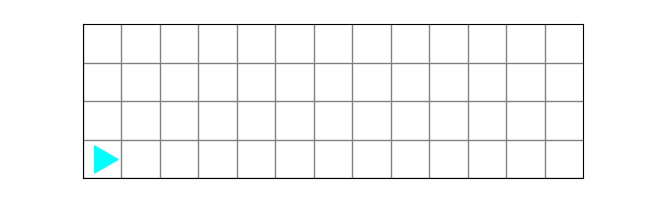Bit needs to draw three trees, with some space between them: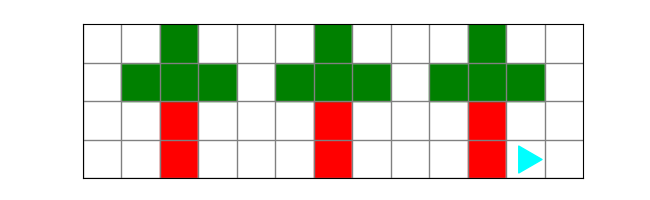Copy and paste your `one_tree()` function and the other helper functions from the previous problem. Be sure to remove the `@Bit.empty_world(5,4)` decorator from the `one_tree()` function.

This problem shows you how using functions makes it easy to re-use code. In future units, you can use an import statement to reuse functions, but for the Bit units you will need to copy and paste.

## 3. Boxes

You are given some code in `boxes.py`. Bit starts in a 3x10 world: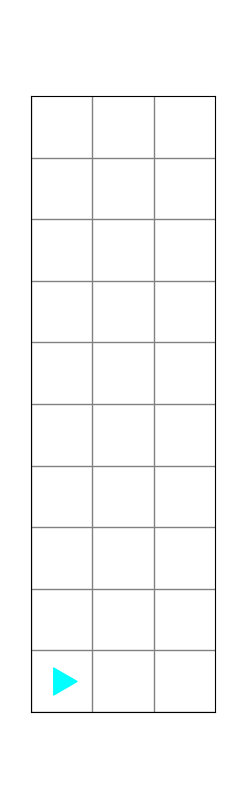Bit needs to stack three boxes, each sitting on a pallet: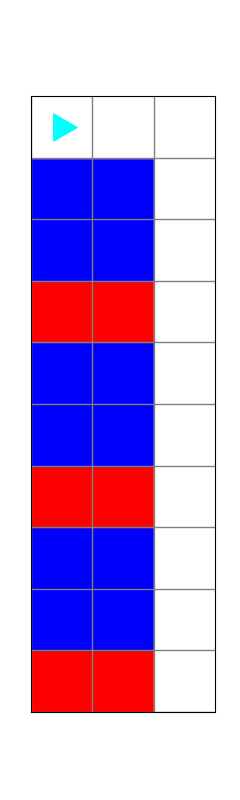The boxes are blue and the pallets are red.

This problem is meant to give you practice with decomposition, so you should write some additional functions and then call them from the `stack_boxes()` function.

## 4. Quilt

You are given some code in `quilt.py`. Bit starts in a 13x3 world: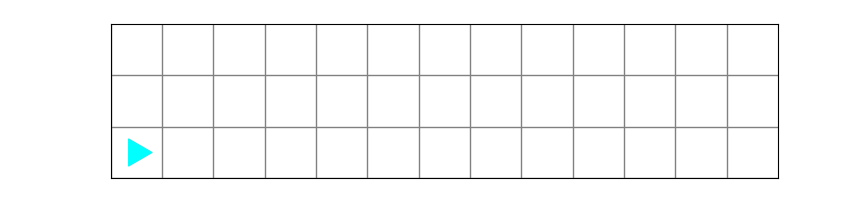Bit needs to draw a quilt pattern: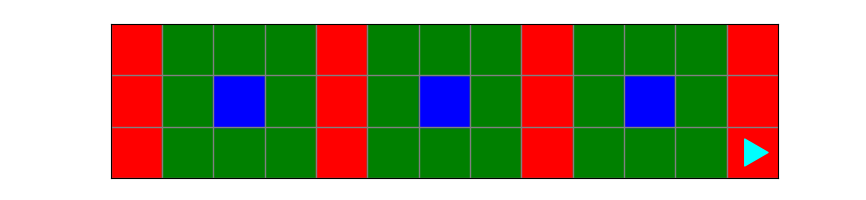This problem is meant to give you practice with decomposition, so you should write some additional functions and then call them from the `make_a_quilt()` function.

`one_tree.py`5
`trees.py`5
`boxes.py`5
`quilt.py`5